## Introduction

Dijkstra 算法是用于求解非负权图单源最短路的经典算法。

• Dijkstra 算法的每个过程是在干什么？
• Dijkstra 算法为什么是正确的？

That is not true.

## Analogy

BFS 同样可以求解最短路，不过它有着更强的限制条件——「边的距离相等」，或者说，「边权为 $1$」.

### Water Filling

• 边权可以 $>1$
• 边权可以为 $0$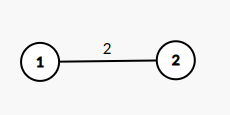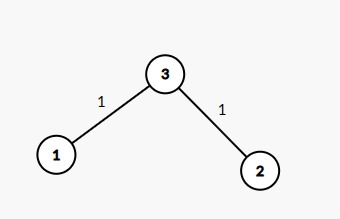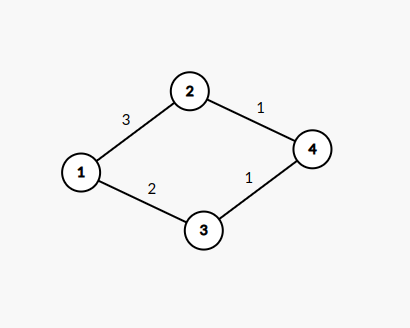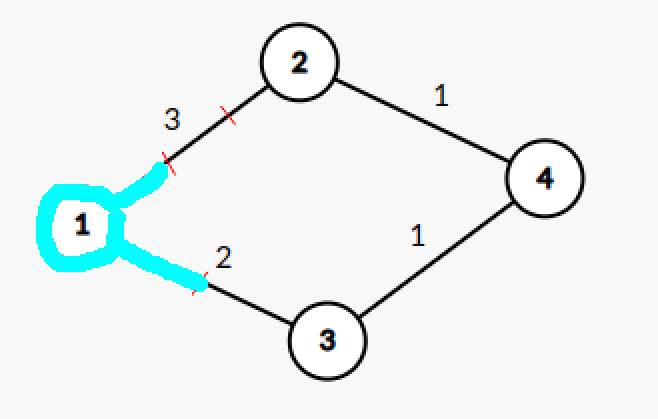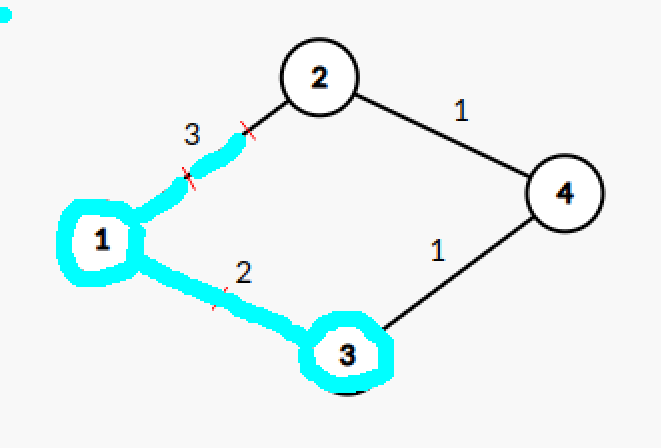#### Solution

• 集合 $\mathbf{T}$ 中的点未被浸泡。
• 每个点首次被浸泡时，水流一定走的是最短路。对应的距离即为最短路距离。
• 下一个被浸泡的点一定从集合 $\mathbf{T}$ 中产生。

• 初始，将起点加入集合 $\mathbf{S}$，将相邻点加入集合 $\mathbf{T}$
• 找出集合 $\mathbf{T}$ 中距离起点最近的点，加入集合 $\mathbf{S}$，将其相邻点加入集合 $\mathbf{T}$
• 重复第二步，直到所有点都加入集合 $\mathbf{S}$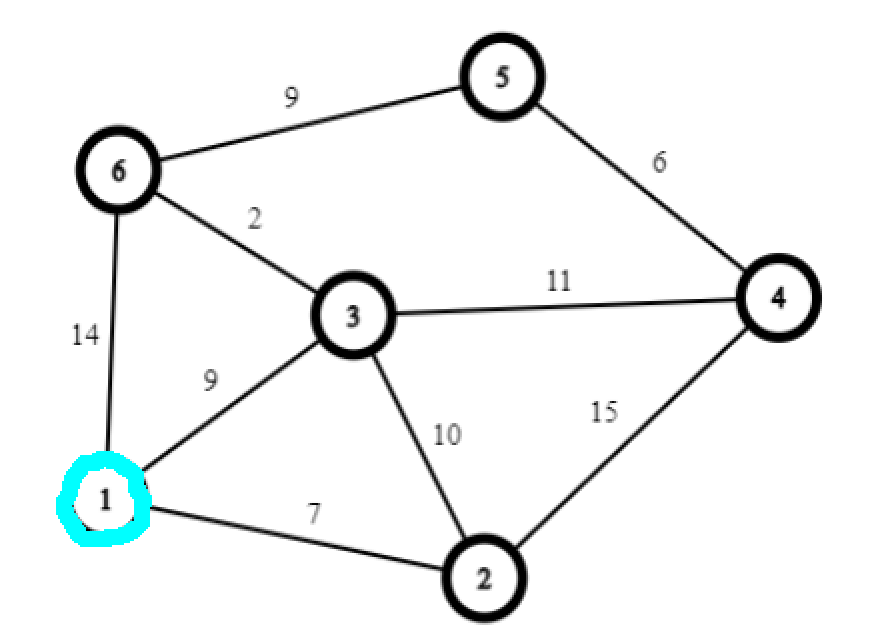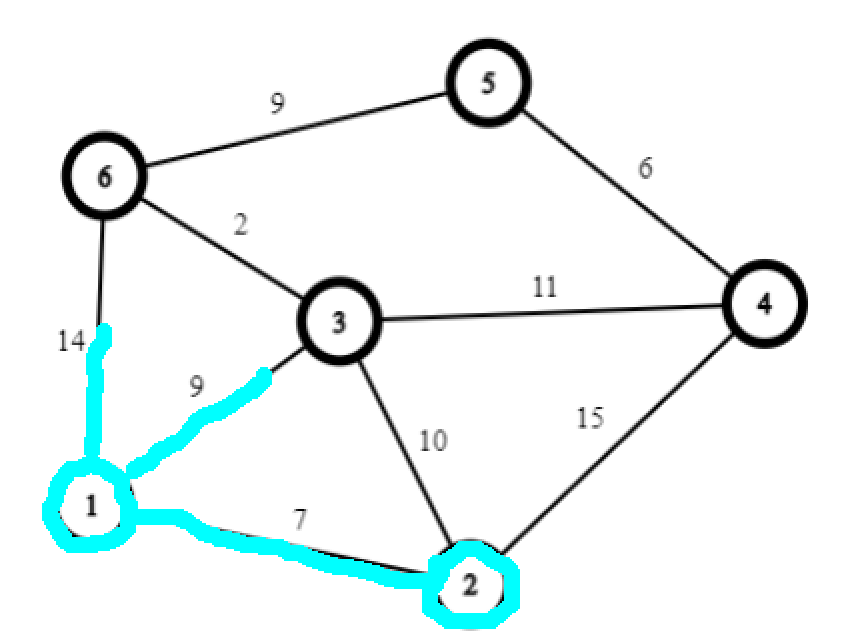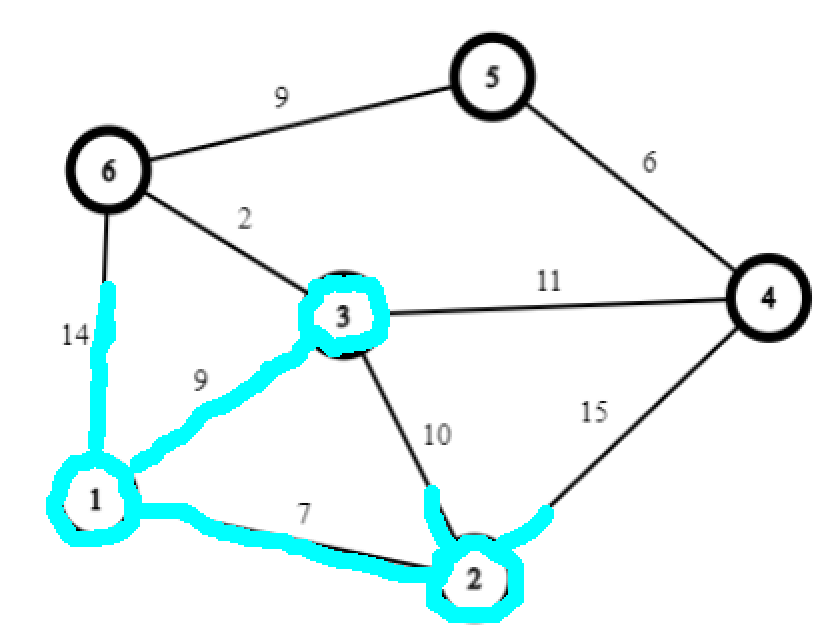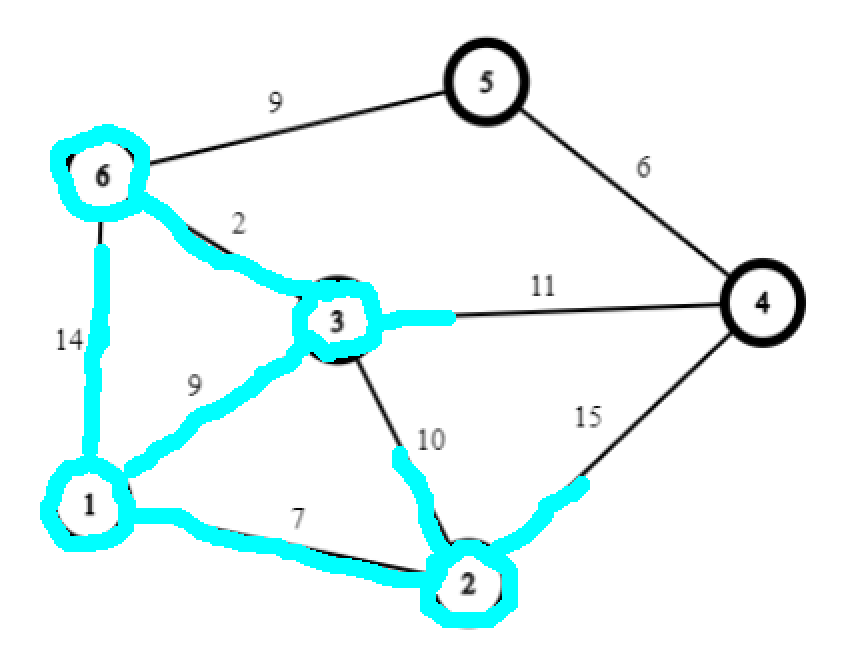## Proof

• 初始，将起点加入集合 $\mathbf{S}$，将相邻点加入集合 $\mathbf{T}$
• 找出集合 $\mathbf{T}$ 中距离起点最近的点，加入集合 $\mathbf{S}$，将其相邻点加入集合 $\mathbf{T}$
• 重复第二步，直到所有点都加入集合 $\mathbf{S}$

$x,y \in \mathbf{S}$，那么 $u,y \in \mathbf{T}$.

PS：这里还能看出 Dijkstra 为什么不能处理负权图。在负权图上，$L < 0$ 会导致 $\operatorname{D}(t) + L \ge \operatorname{D}(u)$ 这个不等式并不成立，核心思想就无效了。

## Implementation

• 初始，将起点加入集合 $\mathbf{S}$，将相邻点加入集合 $\mathbf{T}$
• 找出集合 $\mathbf{T}$ 中距离起点最近的点，加入集合 $\mathbf{S}$，将其相邻点加入集合 $\mathbf{T}$
• 重复第二步，直到所有点都加入集合 $\mathbf{S}$

• 暴力：不使用任何数据结构进行维护，每次 2 操作执行完毕后，直接在集合中暴力寻找最短路长度最小的结点。2 操作总时间复杂度为 $O(m)$，1 操作总时间复杂度为 $O(n^2)$，全过程的时间复杂度为 $O(n^2 + m) = O(n^2)$
• 二叉堆：每成功松弛一条边 $(u,v)$，就将 $v$ 插入二叉堆中（如果 $v$ 已经在二叉堆中，直接修改相应元素的权值即可），1 操作直接取堆顶结点即可。共计 $O(m)$ 次二叉堆上的插入（修改）操作，$O(n)$ 次删除堆顶操作，而插入（修改）和删除的时间复杂度均为 $O(\log n)$，时间复杂度为 $O((n+m) \log n) = O(m\log n)$。
• 优先队列：和二叉堆类似，但使用优先队列时，如果同一个点的最短路被更新多次，因为先前更新时插入的元素不能被删除，也不能被修改，只能留在优先队列中，故优先队列内的元素个数是 $O(m)$ 的，时间复杂度为 $O(m \log m)$。
• Fibonacci 堆：和前面二者类似，但 Fibonacci 堆插入的时间复杂度为 $O(1)$，故时间复杂度为 $O(n\log n + m) = O(n \log n)$，时间复杂度最优。但因为 Fibonacci 堆较二叉堆不易实现，效率优势也不够大 ^1^ ，算法竞赛中较少使用。
• 线段树：和二叉堆原理类似，不过将每次成功松弛后插入二叉堆的操作改为在线段树上执行单点修改，而 1 操作则是线段树上的全局查询最小值。时间复杂度为 $O(m \log n)$。

  1 2 3 4 5 6 7 8 9 10 11 12 13 14 15 16 17 18 19 20 21 22 23 24 25 26 27 28 29 30 31 32 33 34   // C++ Version struct edge { int v, w; }; struct node { int dis, u; bool operator>(const node& a) const { return dis > a.dis; } }; vector e[maxn]; int dis[maxn], vis[maxn]; priority_queue, greater > q; void dijkstra(int n, int s) { memset(dis, 63, sizeof(dis)); dis[s] = 0; q.push({0, s}); while (!q.empty()) { int u = q.top().u; q.pop(); if (vis[u]) continue; vis[u] = 1; for (auto ed : e[u]) { int v = ed.v, w = ed.w; if (dis[v] > dis[u] + w) { dis[v] = dis[u] + w; q.push({dis[v], v}); } } } }